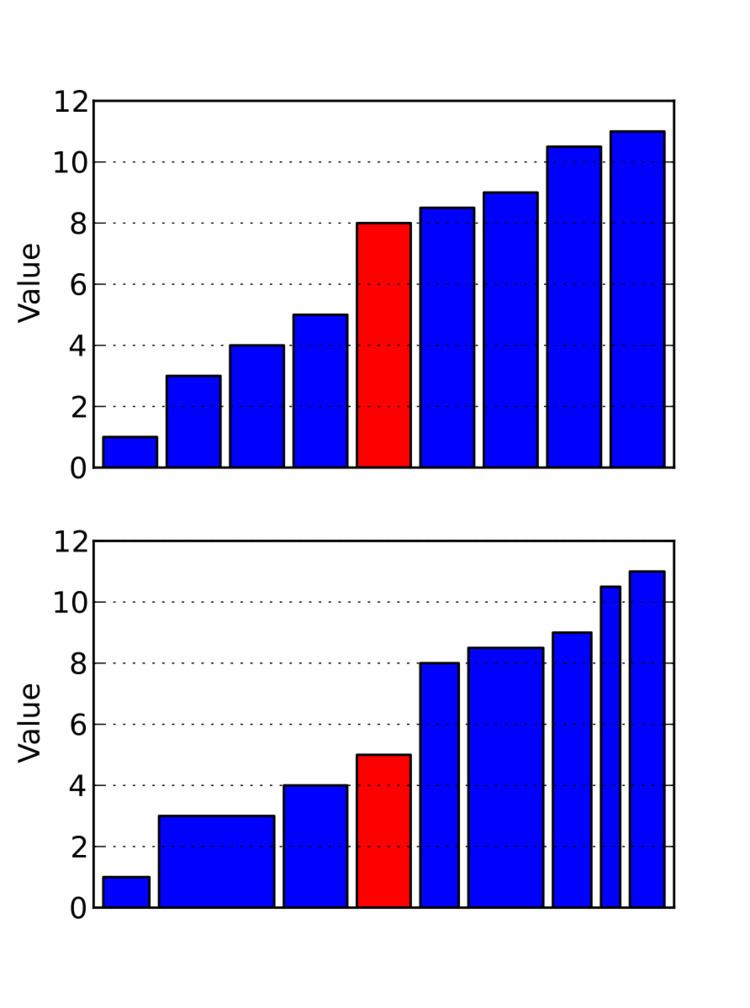# Weighted median

Updated on
Covid-19In statistics, a weighted median of a sample is the 50% weighted percentile. It was first proposed by F. Y. Edgeworth in 1888. Like the median, it is useful as an estimator of central tendency, robust against outliers. It allows for non-uniform statistical weights related to, e.g., varying precision measurements in the sample.

## Definition

For n distinct ordered elements x 1 , x 2 , . . . , x n with positive weights w 1 , w 2 , . . . , w n such that i = 1 n w i = 1 , the weighted median is the element x k satisfying: i = 1 k 1 w i < 1 / 2 and i = k + 1 n w i 1 / 2 . If i = k + 1 n w i = 1 / 2 then the weighted median is x k + x k + m 2 Where x k + m is the next element with non-zero weight. This is a generalization of the standard median which is the weighted median of a set of elements with equal weight.

## Properties

Weighted median of a set partitions that set in two halves such that sum of weights in each partition is as equal as possible.

If weights of all numbers in the set are equal then median is same as weighted median.

When set has even number of elements, we will have lower weighted median and upper weighted median instead of a single element as median.

## Example

Consider the set of numbers { 0.1 ; 0.35 ; 0.05 ; 0.1 ; 0.15 ; 0.05 ; 0.2 } with each number having weights { 0.1 ; 0.35 ; 0.05 ; 0.1 ; 0.15 ; 0.05 ; 0.2 } respectively. The median is 0.1 and the weighted median is 0.2.

## Algorithm

Weighted median can be computed by sorting the set of numbers and finding the smallest numbers which sums to half the weight of total weight. This algorithm takes O ( n log n ) time. There is a better approach to find weighted median using a modified selection algorithm.

Similar Topics